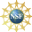# 8. Properties of Curves

## a. Circle

We first look at circular motion represented by the position vector $$\vec{r}(\theta)=(a\cos\theta,a\sin\theta,b)$$ where $$a$$ and $$b$$ are any constants. Here are all the properties of this curve and the plot for the special case with $$a=4$$, $$b=3$$:

Position Vector

$$\vec{r}(\theta)=(4\cos\theta,4\sin\theta,3)$$

Plot

Velocity Vector

$$\vec{v}(\theta)=\dfrac{d\vec{r}}{d\theta} =\langle -4\sin\theta,4\cos\theta,0\rangle$$

Acceleration Vector

$$\vec{a}(\theta)=\dfrac{d\vec{v}}{d\theta} =\langle -4\cos\theta,-4\sin\theta,0\rangle$$

Jerk Vector

$$\vec{j}(\theta)=\dfrac{d\vec{a}}{d\theta} =\langle 4\sin\theta,-4\cos\theta,0\rangle$$

Speed

$$\dfrac{ds}{d\theta}=|\vec{v}| =\sqrt{16\sin^2\theta+16\cos^2\theta}=4$$

Arclength (from $$\theta=\alpha$$ to $$\theta=\beta$$)

$$\displaystyle L=\int_{\alpha}^{\beta} |\vec{v}|\,d\theta =\int_{\alpha}^{\beta} 4\,d\theta =4(\beta-\alpha)$$

Notice this is the product of the radius and the change in angle which is the usual formula for the length of an arc of a circle in radian measure.

Unit Tangent Vector

$$\hat{T}=\dfrac{\vec{v}}{|\vec{v}|} =\dfrac{1}{4}\langle -4\sin\theta,4\cos\theta,0\rangle =\langle -\sin\theta,\cos\theta,0\rangle$$

$$\vec{v}\times\vec{a}= \begin{vmatrix} \hat{\imath} & \hat{\jmath} & \hat{k} \\ -4\sin\theta & 4\cos\theta & 0 \\ -4\cos\theta & -4\sin\theta & 0 \end{vmatrix} =\hat{\imath}(0)-\hat{\jmath}(0)+\hat{k}(16\sin^2\theta+16\cos^2\theta) =\langle 0,0,16\rangle$$

$$|\vec{v}\times\vec{a}|=\sqrt{(0)^2-(0)^2+(16)^2}=16$$

Unit Binormal Vector

$$\hat{B}=\dfrac{\vec{v}\times\vec{a}}{|\vec{v}\times\vec{a}|} =\dfrac{\langle 0,0,16\rangle}{16}=\langle 0,0,1\rangle$$

It is no surprise that the unit binormal is $$\hat{k}$$ since the circle is in a horizontal plane.

Unit Normal Vector

$$\hat{N}=\dfrac{T'(\theta)}{|T'(\theta)|} =\langle -\cos\theta,-\sin\theta,0\rangle$$

It is no surprise that the unit normal is radially inward since the radius vector is perpendicular to the tangent vector.

Curvature

$$\kappa=\dfrac{|\vec{v}\times\vec{a}|}{|\vec{v}|^{3}} =\dfrac{16}{4^{3}}=\dfrac{1}{4}$$

Notice this is the reciprocal of the radius.

Torsion

$$\tau=\dfrac{\vec{v}\times\vec{a}\cdot\vec{j}}{|\vec{v}\times\vec{a}|^2} =\dfrac{\langle 0,0,16\rangle\cdot\langle 4\sin\theta,-4\cos\theta,0\rangle}{16^2} =0$$

No surprise. The plane does not change!

Tangential Acceleration

\begin{aligned} a_{T}&=\vec{a}\cdot\hat{T} =\langle -4\cos\theta,-4\sin\theta,0\rangle \cdot\langle -\sin\theta,\cos\theta,0\rangle\\ &=4\sin\theta\cos\theta-4\sin\theta\cos\theta=0 \\ a_{T}&=\dfrac{d}{d\theta}|\vec{v}|=\dfrac{d}{d\theta}4=0 \end{aligned}

Again no surprise, since the speed is constant and the acceleration is radial and so perpendicular to the tangent vector.

Normal Acceleration

\begin{aligned} a_{N}&=\vec{a}\cdot\hat{N} =\langle -4\cos\theta,-4\sin\theta,0\rangle \cdot\langle -\cos\theta,-\sin\theta,0\rangle \\ &=4\cos^2\theta+4\sin^2\theta=4 \\ a_{N}&=\kappa|\vec{v}|^2= \dfrac{|\vec{v}|^2}{R}=\dfrac{4^2}{4}=4 \end{aligned}

Again no surprise. The acceleration is perpendicular to the tangent vector. So the normal acceleration is the length of the acceleration.

Supported in part by NSF Grant #1123255